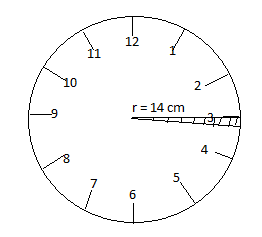Courses
Courses for Kids
Free study material
Free LIVE classes
MoreLIVE
Join Vedantu’s FREE Mastercalss

# The length of the minute hand of a clock is 14 cm. Find the area swept by the minute hand in one minute. [Use$\pi ={}^{22}/{}_{7}$]A. $5.72c{{m}^{2}}$B. $8.01c{{m}^{2}}$C.$10.26c{{m}^{2}}$D. $13.13c{{m}^{2}}$Verified
360.6k+ views
Hint:- Find the degree of the clock for 1 hour. Then find the degree of the clock for 1 minute. Use area of sector to find the area swept by a minute hand in 1 minute.

Complete step-by-step solution -
The minute hand of a clock completes a full circle degree in 1 hour. Consider the clock given below. The degree swept by the minute hand in 1 hour is ${{360}^{\circ }}$ , i.e. the clock resembles circle and the degree of a circle is ${{360}^{\circ }}$ . Thus it will be easier to remember that the degree swept by minute hand in 1 hour is ${{360}^{\circ }}.$We know 1 hour = 60 minutes.
Degree swept by minute hand in 60 minutes = ${{360}^{\circ }}$
$\therefore$Degree swept by minute hand in 1 minute = $\dfrac{360}{60}={{6}^{\circ }}$
$\therefore$Degree swept by the minute hand in 1 minute is ${{6}^{\circ }}$.
Hence, here $\theta = {{6}^{\circ }}$and radius r = 14 cm.
Now we need to find the area swept by the minute hand in 1 minute is equal to the shaded portion in the figure, which can be allocated as a sector. Hence we need to find the area of sector in the shaded region.
Area swept by minute hand = Area of sector.
We know, area of the sector,
\begin{align} & = \dfrac{\theta }{360}\times \pi {{r}^{2}} \\ & = \dfrac{6}{360}\times \dfrac{22}{7}\times {{14}^{2}} \\ & = \dfrac{6}{360}\times \dfrac{22}{7}\times 14\times 14 \\ \end{align}
Cancel out the like terms and simplify it.
Area of sector
$\dfrac{1}{60}\times 22\times 2\times 14\times =\dfrac{11\times 14\times 2}{30} = \dfrac{11\times 7\times 2}{15} = \dfrac{77\times 2}{15} = \dfrac{154}{15} = 10.267c{{m}^{2}}$
$\therefore$Area of sector$= 10.267c{{m}^{2}}$
$\therefore$Area swept by minute hand$= 10.267c{{m}^{2}}$
Hence option C is the correct answer.

Note:- If we were asked to find the area swept in five minutes, then we first find the degree swept by 1 minute and then find the degree swept by 5 minutes.
Degree swept by 1 minute$= {{6}^{\circ }}$
Degree swept by 5 minutes$= {{6}^{\circ }}\times 5={{30}^{\circ }}$
So, area of sector$= \dfrac{30}{360}\times \pi {{r}^{2}} = 51.33c{{m}^{2}} =$area swept by 5 minutes
Similarly, you can find areas swept by the minute hand for other time periods.
Last updated date: 25th Sep 2023
Total views: 360.6k
Views today: 5.60k